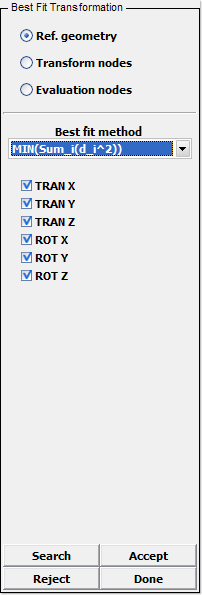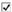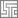# Page 7: BestFit

Home » Knowledge Base » LS-Prepost » Pages » Page7 » Page 7: BestFit

•• Figure 1 – BestFit Interface

## Purpose:

This interface is typically used to gain an objective measure of how much a formed structure deviates from the ideal geometry. The advantage is that this measurement is independent of which nodes are selected for constraining the rigid body motion in the springback analysis.

A collection of nodes, here called Transform nodes, is fitted to geometry defined by a collection of shell elements, here called Ref. geometry, using a rigid body transformation. Another collection of nodes, here called Evaluation nodes, is used to judge how well the Transform nodes fit the geometry. Typically, the Evaluation nodes are a subset of the Transform nodes (or the node sets are identical).

The fit is made such that the Evaluation nodes get as close as possible to the Ref. geometry, and there are two Best fit methods available when performing the fit operation (where d_i is the deviation of evaluation node i from the reference geometry):

• MIN(MAX(d_i))
• Minimize the maximum deviation for all evaluation nodes. This method is much more difficult to evaluate numerically and therefore requires considerably more time to solve.

• MIN(Sum_i(d_i^2))
• Minimize the sum of deviations squared for all evaluation nodes. The objective value reported for this method in the command output area (or Last Command Field) is the sum divided by the number of evaluation nodes which yields a more normalized result.

## Other Controls:

•TRAN X – Activate translation along x in the transformation
•TRAN Y – Activate translation along y in the transformation
•TRAN Z – Activate translation along z in the transformation
•ROT X – Activate rotation about x in the transformation
•ROT Y – Activate rotation about y in the transformation
•ROT Z – Activate rotation about z in the transformation

Search for a best fit

Accept the new node positions

Reject the new node positions

Exit BestFit Interface